### 2. WHAT MIGHT BE GOING ON?

It may seem misguided to put a great deal of energy into exploring models of a small nonzero dark energy density when we have very little idea why the vacuum energy is not as large as the Planck scale. On the other hand, the discovery of dark energy may provide an invaluable clue in our attempts to solve this long-lasting puzzle, giving us reason to redouble our efforts. Explanations of the current acceleration of the universe can be categorized into one of three types:

1. The dark energy is a true cosmological constant, strictly unchanging throughout space and time. The minimum-energy configuration of the universe may have a small but nonvanishing energy density, or we may live in a false vacuum, almost degenerate with the true one but with a small nonzero additional energy.

2. The cosmological constant is zero, but a slowly-varying dynamical component is mimicking a nonzero vacuum energy.

3. Einstein was wrong, and the Friedmann equation does not describe the expansion of the universe.

We briefly examine each of these possibilities in turn.

The simplest interpretation of the dark energy is that we have discovered that the cosmological constant is not quite zero: we are in the lowest energy state possible (or, more properly, that the particles we observe are excitations of such a state) but that energy does not vanish. Although simple, this scenario is perhaps the hardest to analyze without an understanding of the complete cosmological constant problem, and there is correspondingly little to say about such a possibility. As targets to shoot for, various numerological coincidences have been pointed out, which may some day find homes as predictions of an actual theory. For example, the observed vacuum energy scale Mvac = 10-3 eV is related to the 1 TeV scale of low-energy supersymmetry breaking models by a "supergravity suppression factor":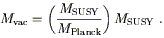(8)

In other words, MSUSY is the geometric mean of Mvac and MPlanck. Unfortunately, nobody knows why this should be the case. In a similar spirit, the vacuum energy density is related to the Planck energy density by the kind of suppression factor familiar from instanton calculations in gauge theories: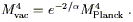(9)

Theorists attempting to build models of a small nonzero vacuum energy must keep in mind the requirement of remaining compatible with some as-yet-undiscovered solution to the cosmological constant problem. In particular, it is certainly insufficient to describe a specific contribution to the vacuum energy which by itself is of the right magnitude; it is necessary at the same time for there to be some plausible reason why the well-known and large contributions from the Standard Model could be suppressed, while the new contribution is not. One way to avoid this problem is to imagine that an unknown mechanism sets the vacuum energy to zero in the state of lowest energy, but that we actually live in a distinct false vacuum state, almost but not quite degenerate in energy with the true vacuum [11, 12, 13]. From an observational point of view, false vacuum energy and true vacuum energy are utterly indistinguishable - they both appear as a strictly constant dark energy density. The issue with such models is why the splitting in energies between the true and false vacua should be so much smaller than all of the characteristic scales of the problem; model-building approaches generally invoke symmetries to suppress some but not all of the effects that could split these levels.

The only theory (if one can call it that) which leads a vacuum energy density of approximately the right order of magnitude without suspicious fine-tuning is the anthropic principle -- the notion that intelligent observers will not witness the full range of conditions in the universe, but only those conditions which are compatible with the existence of such observers. Thus, we do not consider it unnatural that human beings evolved on the surface of the Earth rather than on that of the Sun, even though the surface area of the Sun is much larger, since the conditions are rather less hospitable there. If, then, there exist distinct parts of the universe (whether they be separate spatial regions or branches of a quantum wavefunction) in which the vacuum energy takes on different values, we would expect to observe a value which favored the appearance of life. Although most humans don't think of the vacuum energy as playing any role in their lives, a substantially larger value than we presently observe would either have led to a rapid recollapse of the universe (ifwere negative) or an inability to form galaxies (ifwere positive). Depending on the distribution of possible values of, one can argue that the recently observed value is in excellent agreement with what we should expect [14, 15, 16, 17]. Many physicists find it unappealing to think that an apparent constant of nature would turn out to simply be a feature of our local environment that was chosen from an ensemble of possibilities, although we should perhaps not expect that the universe takes our feelings into account on these matters. More importantly, relying on the anthropic principle involves the invocation of a large collection of alternative possibilities for the vacuum energy, closely spaced in energy but not continuously connected to each other (unless the light scalar fields implied by such connected vacua is very weakly coupled, as it must also be in the quintessence models discussed below). It is by no means an economical solution to the vacuum energy puzzle.

As an interesting sidelight to this issue, it has been claimed that a positive vacuum energy would be incompatible with our current understanding of string theory [18, 19, 20, 21]. At issue is the fact that such a universe eventually approaches a de Sitter solution (exponentially expanding), which implies future horizons which make it impossible to derive a gauge-invariant S-matrix. One possible resolution might involve a dynamical dark energy component such as those discussed in the next section. While few string theorists would be willing to concede that a definitive measurement that the vacuum energy is constant with time would rule out string theory as a description of nature, the possibility of saying something important about fundamental theory from cosmological observations presents an extremely exciting opportunity.

Although the observational evidence for dark energy implies a component which is unclustered in space as well as slowly-varying in time, we may still imagine that it is not perfectly constant. The simplest possibility along these lines involves the same kind of source typically invoked in models of inflation in the very early universe: a scalar field rolling slowly in a potential, sometimes known as "quintessence" [22, 23, 24]. There are also a number of more exotic possibilities, including tangled topological defects and variable-mass particles (see [1, 7] for references and discussion).

There are good reasons to consider dynamical dark energy as an alternative to an honest cosmological constant. First, a dynamical energy density can be evolving slowly to zero, allowing for a solution to the cosmological constant problem which makes the ultimate vacuum energy vanish exactly. Second, it poses an interesting and challenging observational problem to study the evolution of the dark energy, from which we might learn something about the underlying physical mechanism. Perhaps most intriguingly, allowing the dark energy to evolve opens the possibility of finding a dynamical solution to the coincidence problem, if the dynamics are such as to trigger a recent takeover by the dark energy (independently of, or at least for a wide range of, the parameters in the theory).

At the same time, introducing dynamics opens up the possibility of introducing new problems, the form and severity of which will depend on the specific kind of model being considered. The most popular quintessence models feature scalar fieldswith masses of order the current Hubble scale,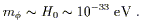(10)

(Fields with larger masses would typically have already rolled to the minimum of their potentials.) In quantum field theory, light scalar fields are unnatural; renormalization effects tend to drive scalar masses up to the scale of new physics. The well-known hierarchy problem of particle physics amounts to asking why the Higgs mass, thought to be of order 1011 eV, should be so much smaller than the grand unification/Planck scale, 1025-1027 eV. Masses of 10-33 eV are correspondingly harder to understand. At the same time, such a low mass implies thatgives rise to a long-range force; even ifinteracts with ordinary matter only through indirect gravitational-strength couplings, searches for fifth forces and time-dependence of coupling constants should have already enabled us to detect the quintessence field .

The need for delicate fine-tunings of masses and couplings in quintessence models is certainly a strike against them, but is not a sufficiently serious one that the idea is not worth pursuing; until we understand much more about the dark energy, it would be premature to rule out any idea on the basis of simple naturalness arguments. One promising route to gaining more understanding is to observationally characterize the time evolution of the dark energy density. In principle any behavior is possible, but it is sensible to choose a simple parameterization which would characterize dark energy evolution in the measurable regime of relatively nearby redshifts (order unity or less). For this purpose it is common to imagine that the dark energy evolves as a power law with the scale factor: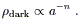(11)

Even if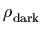is not strictly a power law, this ansatz can be a useful characterization of its effective behavior at low redshifts. It is common to define an equation-of-state parameter relating the energy density to the pressure,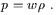(12)

Using the equation of energy-momentum conservation,(13)

a constant exponent n of (11) implies a constant w with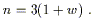(14)

As n varies from 3 (matter) to 0 (cosmological constant), w varies from 0 to -1. (Imposing mild energy conditions implies that | w|1 ; however, models with w < - 1 are still worth considering .)

Some limits from supernovae and large-scale structure from  are shown in Figure (2). These constraints apply to the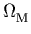-w plane, under the assumption that the universe is flat (+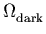= 1). We see that the observationally favored region features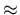0.35 and an honest cosmological constant, w = - 1. However, there is plenty of room for alternatives; one of the most important tasks of observational cosmology will be to reduce the error regions on plots such of these to pin down precise values of these parameters.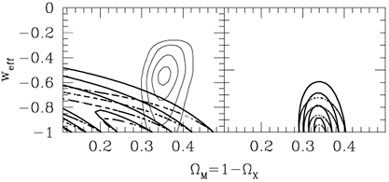Figure 2. Limits on the equation-of-state parameter w in a flat universe, where+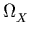= 1. The left-hand panel shows limits from supernova data (lower left corner) and large-scale structure (ellipses); the right-hand panel shows combined constraints. From .

To date, many investigations have considered scalar fields with potentials that asymptote gradually to zero, of the form e1 /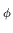or 1/. These can have cosmologically interesting properties, including "tracking" behavior that makes the current energy density largely independent of the initial conditions ; they can also be derived from particle-physics models, such as the dilaton or moduli of string theory. They do not, however, provide a solution to the coincidence problem, as the era in which the scalar field begins to dominate is still set by finely-tuned parameters in the theory. There have been two scalar-field models which come closer to being solutions: "k-essence", and oscillating dark energy. The k-essence idea  does not put the field in a shallow potential, but rather modifies the form of the kinetic energy. We imagine that the Lagrange density is of the form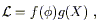(15)

where X =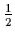()2 is the conventional kinetic term. For certain choices of the functions f () and g(X), the k-essence field naturally tracks the evolution of the total radiation energy density during radiation domination, but switches to being almost constant once matter begins to dominate. In such a model the coincidence problem is explained by the fact that matter/radiation equality was a relatively recent occurrence (at least on a logarithmic scale). The oscillating models  involve ordinary kinetic terms and potentials, but the potentials take the form of a decaying exponential with small perturbations superimposed: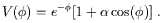(16)

On average, the dark energy in such a model will track that of the dominant matter/radiation component; however, there will be gradual oscillations from a negligible density to a dominant density and back, on a timescale set by the Hubble parameter. Consequently, in such models the acceleration of the universe is just something that happens from time to time. Unfortunately, in neither the k-essence models nor the oscillating models do we have a compelling particle-physics motivation for the chosen dynamics, and in both cases the behavior still depends sensitively on the precise form of parameters and interactions chosen. Nevertheless, these theories stand as interesting attempts to address the coincidence problem by dynamical means.

Rather than constructing models on the basis of cosmologically interesting dynamical properties, we may take the complementary route of considering which models would appear most sensible from a particle-physics point of view, and then exploring what cosmological properties they exhibit. An acceptable particle physics model of quintessence would be one in which the scalar mass was naturally small and its coupling to ordinary matter was naturally suppressed. These requirements are met by Pseudo-Nambu-Goldstone bosons (PNGB's) , which arise in models with approximate global symmetries of the form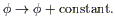(17)

Clearly such a symmetry should not be exact, or the potential would be precisely flat; however, even an approximate symmetry can naturally suppress masses and couplings. PNGB's typically arise as the angular degrees of freedom in Mexican-hat potentials that are "tilted" by a small explicitly symmetry breaking, and the PNGB potential takes on a sinusoidal form: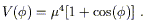(18)

As a consequence, there is no easily characterized tracking or attractor behavior; the equation of state parameter w will depend on both the potential and the initial conditions, and can take on any value from -1 to 0 (and in fact will change with time). We therefore find that the properties of models which are constructed by taking particle-physics requirements as our primary concern appear quite different from those motivated by cosmology alone. The lesson to observational cosmologists is that a wide variety of possible behaviors should be taken seriously, with data providing the ultimate guidance.

Given the uncomfortable tension between observational evidence for dark energy on one hand and our intuition for what seems natural in the context of the standard cosmological model on the other, there is an irresistible temptation to contemplate the possibility that we are witnessing a breakdown of the Friedmann equation of conventional general relativity (GR) rather than merely a novel source of energy. Alternatives to GR are highly constrained by tests in the solar system and in binary pulsars; however, if we are contemplating the space of all conceivable alternatives rather than examining one specific proposal, we are free to imagine theories which deviate on cosmological scales while being indistinguishable from GR in small stellar systems. Speculations along these lines are also constrained by observations: any alternative must predict the right abundances of light elements from Big Bang nucleosynthesis (BBN), the correct evolution of a sensible spectrum of primordial density fluctuations into the observed spectrum of temperature anisotropies in the Cosmic Microwave Background and the power spectrum of large-scale structure, and that the age of the universe is approximately twelve billion years. Of these phenomena, the sharpest test of Friedmann behavior comes from BBN, since perturbation growth depends both on the scale factor and on the local gravitational interactions of the perturbations, while a large number of alternative expansion histories could in principle give the same age of the universe. As an example, Figure (3) provides a graphical representation of alternative expansion histories in the vicinity of BBN ( HBBN ~ 0.1 sec-1) which predict the same light element abundances as the standard picture . The point of this figure is that expansion histories which are not among the family portrayed, due to differences either in the slope or the overall normalization, will not give the right abundances. So it is possible to find interesting nonstandard cosmologies which are consistent with the data, but they describe a small set in the space of all such alternatives.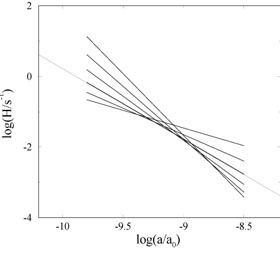Figure 3. The range of allowed evolution histories during Big Bang nucleosynthesis (between temperatures of 1 MeV to 50 keV), expressed as the behavior of the Hubble parameter H =/a as a function of a. Changes in the normalization of H can be compensated by a change in the slope while predicting the same abundances of 4He, 2D, and 7Li. The extended thin line represents the standard radiation-dominated Friedmann universe model. From .

Rather than imagining that gravity follows the predictions of standard GR in localized systems but deviates in cosmology, another approach would be to imagine that GR breaks down whenever the gravitational field becomes (in some sense) sufficiently weak. This would be unusual behavior, as we are used to thinking of effective field theories as breaking down at high energies and small length scales, but being completely reliable in the opposite regime. On the other hand, we might be ambitious enough to hope that an alternative theory of gravity could explain away not only the need for dark energy but also that for dark matter. It has been famously pointed out by Milgrom  that the observed dynamics of galaxies only requires the introduction of dark matter in regimes where the acceleration due to gravity (in the Newtonian sense) falls below a certain fixed value,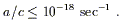(19)

Meanwhile, we seem to need to invoke dark energy when the Hubble parameter drops approximately to its current value,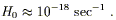(20)

A priori, there seems to be little reason to expect that these two phenomena should be characterized by timescales of the same order of magnitude; one involves the local dynamics of baryons and non-baryonic dark matter, while the other involves dark energy and the overall matter density (although see  for a suggested explanation). It is natural to wonder whether this is simply a numerical coincidence, or the reflection of some new underlying theory characterized by a single dimensionful parameter. To date, nobody has succeeded in inventing a theory which comes anything close to explaining away both the dark matter and dark energy in terms of modified gravitational dynamics. Given the manifold successes of the dark matter paradigm, from gravitational lensing to structure formation to CMB anisotropy, is seems a good bet to think that this numerical coincidence is simply an accident. Of course, given the incredible importance of finding a successful alternative theory, there seems to be little harm in keeping an open mind.

It was mentioned above, and bears repeating, that modified-gravity models do not hold any unique promise for solving the coincidence problem. At first glance we might hope that an alternative to the conventional Friedmann equation might lead to a naturally occurring acceleration at all times; but a moment's reflection reveals that perpetual acceleration is not consistent with the data, so we still require an explanation for why the acceleration began recently. In other words, the observations seem to be indicating the importance of a fixed scale at which the universe departs from ordinary matter domination; if we are fortunate we will explain this scale either in terms of combinations of other scales in our particle-physics model or as an outcome of dynamical processes, while if we are unfortunate it will have to be a new input parameter to our theory. In either case, finding the origin of this new scale is the task for theorists and experimenters in the near future.